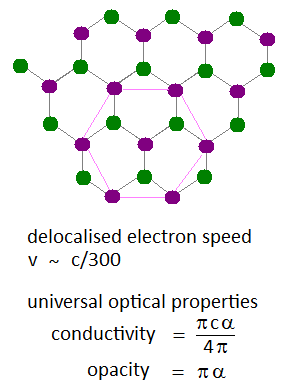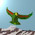## Thursday, November 4, 2010

### Compulsory Graphene Post

Today's excellent local seminar, by Uli Zuelicke, discussed the relativistic nature of delocalised electron systems in graphene. There excitations are essentially massless at certain critical points, for which the lack of a gap between conduction and valence bands creates a light cone like surface. We consider a graphene lattice diagram with two hexagonal site colours, much as for benzene molecules.The optical properties of graphene exhibit universal behaviour, in terms of the fine structure constant $\alpha$. Single atom layers are rather transparent, but some absorption defines an opacity which is a factor of $4 \pi$ different from the universal optical conductivity (at high energies). The stepwise shift in opacity has been measured for a number of atomic layers.1.Ah, so I am not the first to notice this link to 'AdS/CFT' results for quark gluon plasmas etc. Unfortunately, my colleagues here are not thinking about these things ...

2.From your link: All charges comes from dissipation, entropy. Like a quantized entropy?
The strong force vanish first at high temperatures?

What about your graphene link that showed the graphene was ruled only by alpha and pi, nothing at all from the graphene. It is totally from the vacuum field alone? Reminds me of induced metabolism in life.

3.The real fun starts with topological insulators. Think of this as the case when the number of dirac cones is odd, leading to topologically protected metallic boundary states. Take a look at the slides by S. Ryu: Topological Insulators/Superconductors and D-branes.

Kitaev classified many of the topological insulators and superconductors by their homotopy groups using Bott periodicity (arXiv:0901.2686). Essentially, each system he considers has a corresponding non-linear sigma model, with a given target space given by a coset G/H. By computing the homotopy groups (pi_n(G/H)) for each coset (for a given n), one can determine if there exists a topological insulator or superconductor in a given dimension. The vanishing of the nth homotopy group means there is no topological insulator or superconductor present in the corresponding dimension.

The relation to supergravity is pretty straightforward mathematically, as a given supergravity theory can be viewed a non-linear sigma model with scalar fields taking values in a target space G/H, where G is the nonlinear bosonic symmetry and H (R-symmetry group) is a maximal compact subgroup of G. Relating a given supergravity theory to a condensed matter system amounts to matching up their non-linear sigma models and target spaces. This gives provides a theoretical 'bridge' between the two subjects, allowing one to re-cast the condensed matter system in the language of AdS/CFT and D-branes for instance.

4.Great, kneemo! Nice to hear from you.

Note: Only a member of this blog may post a comment.